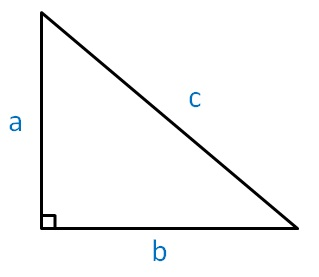Pythagorean triplets

Chapter 6 Class 8 Squares and Square Roots
Concept wise

A Pythagorean Triplet has 3 numbers a, b, c

and

a 2 + b 2 = c 2

Thus, we say that (a, b, c) are Pythagorean triplet

Note : This a, b, c are sides of a right triangleThe most common Pythagorean Triplets are

• 3, 4, 5
• 5, 12, 13
• 7, 24, 25
• 8, 15, 17

Let’s see how we check them

#### For Numbers 3, 4, 5

3 2 + 4 2 = 9+16

= 25

= 5 2

∴ 3 2 +4 2 =5 2

Thus, 3, 4, 5 are Pythagoras Triplets.

#### For Number  5, 12, 13

5 + 12 2 = 25 + 144

= 169

= 13 2

Thus, 5, 12, 13 are Pythagorean Triplets.

#### In General

(2m) + (m - 1) 2 = (m 2 + 1) 2

Here,

First number = 2m

Second number = m 2 - 1

Third number = m 2 + 1

from Pythagoras Triplets.

Note:

If we know that 3, 4, 5 are Pythagorean Triplets

Then,

3 × 2 = 6

4 × 2 = 8

5 × 2 = 10

So, (6, 8, 10) will also be a Pythagorean Triplet

Similarly, if we multiply by 3

(9, 12, 15) is also a Pythagorean Triplet

And, more generally, if we multiply by any number k

(3k, 4k, 5k) will also be a Pythagorean Triplet

Get live Maths 1-on-1 Classs - Class 6 to 12